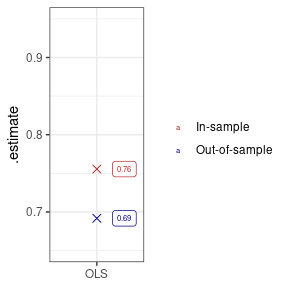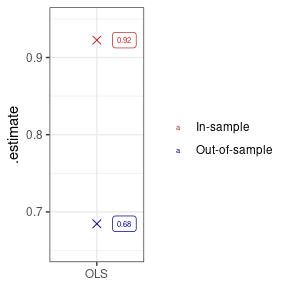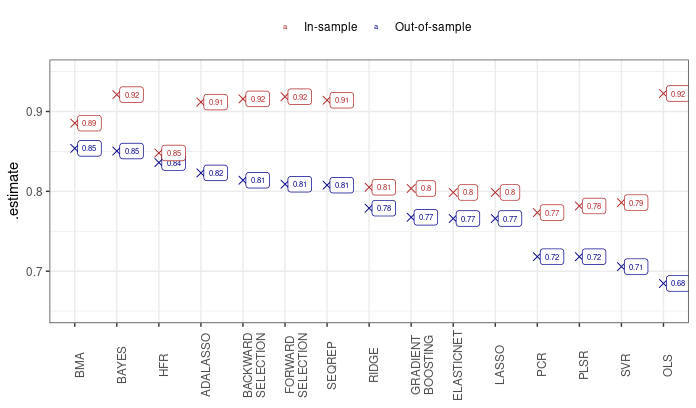# Predicting Boston House Prices

library(tidyverse) # Data wrangling
library(tidyfit)   # Auto-ML modeling

The Boston house prices data set (MASS::Boston) presents a popular test case for regression algorithms. In this article, I assess the relative performance of 15 different linear regression techniques using tidyfit::regress.

Let’s begin by loading the data, and splitting it into equally sized training and test sets:

data <- MASS::Boston

# For reproducibility
set.seed(123)
ix_tst <- sample(1:nrow(data), round(nrow(data)*0.5))

data_trn <- data[-ix_tst,]
data_tst <- data[ix_tst,]

as_tibble(data)
#> # A tibble: 506 × 14
#>       crim    zn indus  chas   nox    rm   age   dis   rad   tax ptratio black
#>      <dbl> <dbl> <dbl> <int> <dbl> <dbl> <dbl> <dbl> <int> <dbl>   <dbl> <dbl>
#>  1 0.00632  18    2.31     0 0.538  6.58  65.2  4.09     1   296    15.3  397.
#>  2 0.0273    0    7.07     0 0.469  6.42  78.9  4.97     2   242    17.8  397.
#>  3 0.0273    0    7.07     0 0.469  7.18  61.1  4.97     2   242    17.8  393.
#>  4 0.0324    0    2.18     0 0.458  7.00  45.8  6.06     3   222    18.7  395.
#>  5 0.0690    0    2.18     0 0.458  7.15  54.2  6.06     3   222    18.7  397.
#>  6 0.0298    0    2.18     0 0.458  6.43  58.7  6.06     3   222    18.7  394.
#>  7 0.0883   12.5  7.87     0 0.524  6.01  66.6  5.56     5   311    15.2  396.
#>  8 0.145    12.5  7.87     0 0.524  6.17  96.1  5.95     5   311    15.2  397.
#>  9 0.211    12.5  7.87     0 0.524  5.63 100    6.08     5   311    15.2  387.
#> 10 0.170    12.5  7.87     0 0.524  6.00  85.9  6.59     5   311    15.2  387.
#> # … with 496 more rows, and 2 more variables: lstat <dbl>, medv <dbl>

The aim is to predict the median value of owner-occupied homes ('medv' in the data set) using the remaining columns.1 We will assess performance using $$R^2$$, which allows intuitive comparisons of in-sample and out-of-sample fit.

## A simple regression

To establish a baseline, we fit an OLS regression model and examine the test and training set R-squared. The predict function can be used to obtain predictions, and yardstick is used to assess performance. The regression is estimated using tidyfit::regress. tidyfit::m is a generic model wrapper that is capable of fitting a large number of different algorithms:

model_frame <- data_trn %>%
regress(medv ~ ., OLS = m("lm"))

Let’s create a helper function to assess performance. The helper function wraps predict and prepares in and out-of-sample performance stats:

assess <- function(model_frame, data_trn, data_tst) {

oos <- model_frame %>%
predict(data_tst) %>%
mutate(type = "Out-of-sample") %>%
arrange(desc(.estimate))

is <- model_frame %>%
predict(data_trn) %>%
mutate(type = "In-sample")

return(bind_rows(oos, is))

}

plotter <- function(df) {
df %>%
mutate(lab = round(.estimate, 2)) %>%
mutate(model = str_wrap(model, 12)) %>%
mutate(model = factor(model, levels = unique(.\$model))) %>%
ggplot(aes(model, .estimate)) +
geom_point(aes(color = type), size = 2.5, shape = 4) +
geom_label(aes(label = lab, color = type), size = 2, nudge_x = 0.35) +
theme_bw() +
scale_color_manual(values = c("firebrick", "darkblue")) +
theme(legend.title = element_blank(),
axis.title.x = element_blank()) +
coord_cartesian(ylim = c(0.65, 0.95))
}

Now we plot the resulting metrics:

model_frame %>%
assess(data_trn, data_tst) %>%
plotterThe in-sample fit of the regression is naturally better than out-of-sample, but the difference is not large. The results can be improved substantially by adding interaction terms between features. This leads to a vast improvement in in-sample fit (again, this is a logical consequence of including a large number of regressors), but leads to no improvement in out-of-sample fit, suggesting substantial overfit:

model_frame <- data_trn %>%
regress(medv ~ .*., OLS = m("lm"))

model_frame %>%
assess(data_trn, data_tst) %>%
plotter## Regularized regression estimators

Regularization controls overfit by introducing parameter bias in exchange for a reduction in estimator variance. A large number of approaches to regularization exist that can be summarized in at least 5 categories:

1. Best subset selection
2. L1/L2 penalized regression
3. Latent factor regression
4. Other machine learning
5. Bayesian regression

tidyfit includes estimates from each of these categories, as summarized below:

Best subset L1/L2 penalized Latent factors Machine Learning Bayesian
Exhaustive search (leaps) Lasso (glmnet) Principal components regression (pls) Gradient Boosting (mboost) Bayesian regression (arm)
Forward selection (leaps) Ridge (glmnet) Partial least squares (pls) Hierarchical feature regression (hfr) Bayesian model averaging (BMS)
Backward selection (leaps) ElasticNet (glmnet) Support vector regression (e1071)
Sequential Replacement (leaps) Adaptive Lasso (glmnet)

In the next code chunk, 15 of the available regression techniques are fitted to the data set. Hyperparameters are optimized using a 10-fold cross validation powered by rsample in the background. As a practical aside, it is possible to set a future plan to ensure hyperparameter tuning is performed on a parallelized backend.2 This is done using plan(multisession(workers = n_workers)), where n_workers is the number of available cores. Furthermore, a progress bar could be activated using progressr::handlers(global=TRUE).

model_frame_all <- data_trn %>%
regress(medv ~ .*.,
OLS = m("lm"),
BAYES = m("bayes"),
BMA = m("bma", iter = 5000),
SEQREP = m("subset", method = "seqrep", IC = "AIC"),
FORWARD SELECTION = m("subset", method = "forward", IC = "AIC"),
BACKWARD SELECTION = m("subset", method = "backward", IC = "AIC"),
LASSO = m("lasso"),
RIDGE = m("ridge"),
ELASTICNET = m("enet"),
PCR = m("pcr"),
PLSR = m("plsr"),
HFR = m("hfr"),
GRADIENT BOOSTING = m("boost"),
SVR = m("svm"),
.cv = "vfold_cv", .cv_args = list(v = 10))

Let’s use yardstick again to calculate in-sample and out-of-sample performance statistics. The models are arranged descending order of test set performance:

eval_frame <- model_frame_all %>%
assess(data_trn, data_tst)
eval_frame %>%
plotter +
theme(legend.position = "top",
axis.text.x = element_text(angle = 90))The Bayesian regression techniques achieve the highest out-of-sample performance, followed by the hierarchical feature regression. The subset selection algorithms achieve relatively good results, while the penalized and latent factor regressions perform worst. All methods improve on the OLS results.

## A glimpse at the backend

tidyfit makes it exceedingly simple to fit different regularized linear regressions. The package ensures that the input and output of the modeling engine, tidyfit::m are standardized and model results are comparable. For instance, whenever necessary, features are standardized with the coefficients transformed back to the original scale. Hyperparameter grids are set to reasonable starting values and can be passed manually to tidyfit::m.

The package further includes a tidyfit::classify method that assumes a binomial or multinomial response. Many of the methods implemented can handle Gaussian and binomial responses. Furthermore, the tidyfit::regress and tidyfit::classify methods seamlessly integrate with grouped tibbles, making it extremely powerful to estimate a large number of regressions for different data groupings. Groups as well as response distributions are automatically respected by the predict method.

1. Have a look at ?MASS::Boston for definitions of the features.↩︎

2. Parallelizing happens at the level of cross validation slices, so always consider if the overhead of parallel computation is worth it. For instance, parallelizing will likely be very useful with .cv = "loo_cv" but can be less useful with .cv = "vfold_cv".↩︎